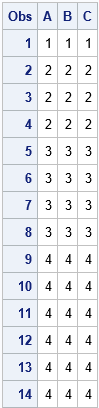In SAS/IML programs, a common task is to write values in a matrix to a SAS data set. For some programs, the values you want to write are in a matrix and you use the CREATE FROM/APPEND FROM syntax to create the data set, as follows:

```proc iml; X = {1 2 3, 4 5 6, 7 8 9, 10 11 12}; create MyData from X[colname={'A' 'B' 'C'}]; /* create data set and variables */ append from X; /* write all rows of X */ close; /* close the data set */```

In other programs, the results are computed inside an iterative DO loop. If you can figure out how many observations are generated inside the loop, it is smart to allocate room for the results prior to the loop, assign the rows inside the loop, and then write to a data set after the loop.

However, sometimes you do not know in advance how many results will be generated inside a loop. Examples include certain kinds of simulations and algorithms that iterate until convergence. An example is shown in the following program. Each iteration of the loop generates a different number of rows, which are appended to the Z matrix. If you do not know in advance how many rows Z will eventually contain, you cannot allocate the Z matrix prior to the loop. Instead, a common technique is to use vertical concatenation to append each new result to the previous results, as follows:

```/* sometimes it is hard to determine in advance how many rows are in the final result */ free Z; do n = 1 to 4; k = n + floor(n/2); /* number of rows */ Y = j(k , 3, n); /* k x 3 matrix */ Z = Z // Y; /* vertical concatenation of results */ end; create MyData2 from Z[colname={'A' 'B' 'C'}]; /* create data set and variables */ append from Z; /* write all rows */ close; /* close the data set */```

Concatenation within a loop tends to be inefficient. As I like to say, "friends don't let friends concatenate results inside a loop!"

If your ultimate goal is to write the observations to a data set, you can write each sub-result to the data set from inside the DO loop! The APPEND FROM statement writes whatever data are in the specified matrix, and you can call the APPEND FROM statement multiple times. Each call will write the contents of the matrix to the open data set. You can update the matrix or even change the number of rows in the matrix. For example, the following program opens the data set prior to the DO loop, appends to the data set multiple times (each time with a different number of rows), and then closes the data set after the loop ends.

```/* alternative: create data set, write to it during the loop, then close it */ Z = {. . .}; /* tell CREATE stmt that data will contain three numerical variables */ create MyData3 from Z[colname={'A' 'B' 'C'}]; /* open before the loop. The TYPE of the variables are known. */ do n = 1 to 4; k = n + floor(n/2); /* number of rows */ Z = j(k , 3, n); /* k x 3 matrix */ append from Z; /* write each block of data */ end; close; /* close the data set */```

The following output shows the contents of the MyData3 data set, which is identical to the MyData2 data set:Notice that the CREATE statement must know the number and type (numerical or character) of the data set variables so that it can set up the data set for writing. If you are writing character variables, you also need to specify the length of the variables. I typically use missing values to tell the CREATE statement the number and type of the variables. These values are not written to the data set. It is the APPEND statement that writes data.

I previously wrote about this technique in the article "Writing data in chunks," which was focused on writing large data set that might not fit into RAM. However, the same technique is useful for writing data when the total number of rows is not known until run time. I also use it when running simulations that generate multivariate data. This technique provides a way to write data from inside a DO loop and to avoid concatenating matrices within the loop.

The post Write to a SAS data set from inside a SAS/IML loop appeared first on The DO Loop.